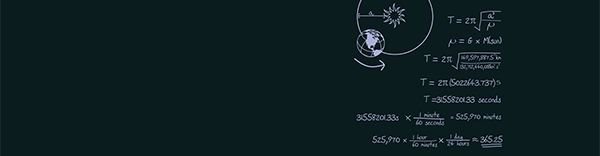# OCR A Level Mathematics

## Tuition Plan: Core Mathematics 1 (Unit C1)

### Written exam – May/June – 1 hour 30 min 16.67 % of OCR A Level Mathematics

#### Overview of assessment

The unit C1 is assessed through a 1.5 hour written examination paper which is set and marked by OCR.

The total number of marks is 72.

## Tuition Plan: Core Mathematics 2 (Unit C2)

### Written exam – May/June – 1 hour and 30 min 16.67 % of OCR A Level Mathematics

#### Overview of assessment

The unit is assessed through a 1.5 hour written examination paper, set and marked by OCR.

The total number of marks is 72.

## Tuition Plan: Core Mathematics 3 (Unit C3)

### Written exam – May/June – 1 hour and 30 min 16.67 % of OCR A Level Mathematics

#### Overview of assessment

The unit is assessed through a 1.5 hour written examination paper, set and marked by OCR.

The total number of marks is 72.

## Tuition Plan: Core Mathematics 4 (Unit C4)

### Written exam – May/June – 1 hour and 30 min 16.67 % of OCR A Level Mathematics

#### Overview of assessment

The unit is assessed through a 1.5 hour written examination paper, set and marked by OCR.

The total number of marks is 72.

## Tuition Plan: OCR optional units

### 16.67 % of OCR A Level Mathematics

#### Overview of assessment

The optional unit is assessed through 1.5 hour written examination paper. Students are allowed to choose one of three options: M1, S1 or D1

The total number of marks is 72.